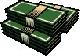Financial Terms Nominal

# Definition of Nominal## Nominal

In name only. Differences in compounding cause the nominal rate to differ from the effective
interest rate. Inflation causes the purchasing power of money to differ from one time to another.

## Nominal

Measured in money terms, in current rather than real dollars. Contrast with real.

# Related Terms:

## Deferred nominal life annuity

A monthly fixed-dollar payment beginning at retirement age. It is nominal
because the payment is fixed in dollar amount at any particular time, up to and including retirement.

## Nominal annual rate

An effective rate per period multiplied by the number of periods in a year.

## Nominal cash flow

A cash flow expressed in nominal terms if the actual dollars to be received or paid out are given.

## Nominal exchange rate

The actual foreign exchange quotation in contrast to the real exchange rate that has

## Nominal interest rate

The interest rate unadjusted for inflation.

## Nominal price

Price quotations on futures for a period in which no actual trading took place.## Nominal Interest Rate

The rate of interest quoted, or stated, to be paid on a security

## nominal interest rate

Rate at which money invested grows.

## Exchange Rate, Nominal

The price of one currency in terms of another, in this book defined as number of units of foreign currency per dollar.

## Interest Rate, Nominal

Payment for the use of borrowed funds, measured as a percentage per year of these funds.

## Nominal Interest Rate

The contracted, or stated, interest rate, undeflated for price level changes.

## Fisher effect

A theory that nominal interest rates in two or more countries should be equal to the required real
rate of return to investors plus compensation for the expected amount of inflation in each country.

## Guaranteed insurance contract

A contract promising a stated nominal interest rate over some specific time
period, usually several years.

## Real interest rate

The rate of interest excluding the effect of inflation; that is, the rate that is earned in terms
of constant-purchasing-power dollars. Interest rate expressed in terms of real goods, i.e. nominal interest rate

## Face Value

The nominal value of a security. Also called the par value.## Par Value

nominal value of a security. Same as face value.

## Coupon rate

The nominal interest rate that the issuer promises to pay the

## Yield

a. Measure of return on an investment, stated as a percentage of price.
Yield can be computed by dividing return by purchase price, current market
value, or other measure of value.
b. Income from a bond expressed as an
annualized percentage rate.
c. The nominal annual interest rate that gives a
future value of the purchase price equal to the redemption value of the security.
Any coupon payments determine part of that yield.

## international Fisher effect

Theory that real interest rates in all countries should be equal, with differences in nominal rates reflecting differences in expected inflation.

## Deflator

A price index used to deflate a nominal value to a real value by dividing the nominal value by the price deflator.

## Exchange Rate, Real

The nominal exchange rate corrected for price level differences.

## GDP Deflator

Price index used to deflate nominal GDP to real GDP by dividing nominal GDP by the GDP deflator.

## Interest Rate, Real

nominal interest rate less expected inflation.

## Money Rate of Interest

See interest rate, nominal.

## Quantity Theory of Money

Theory that velocity is constant, and so a change in money supply will change nominal income by the same percentage. Formalized by the equation Mv = PQ.

## Real

Measured in base year, or constant, dollars. Contrast with nominal.

## Real GDP

GDP expressed in base-year dollars, calculated by dividing nominal GDP by a price index.

## Real Income

Income expressed in base-year dollars, calculated by dividing nominal income by a price index.

## Velocity

The number of times during a year that the money supply turns over in supporting that year's economic activity, measured as the ratio of nominal income to the money supply.

## Face Value

The nominal value which appears on the face of a document recording an entitlement, generally an amount of money that has to be repaid on the maturity of a debt instrument.